# Aggregate points

Aggregate Points summarizes points into polygons or bins. The boundaries from the polygons or bins are used to collect the points within each area and use them to calculate statistics. The result always contains the count of points within each area.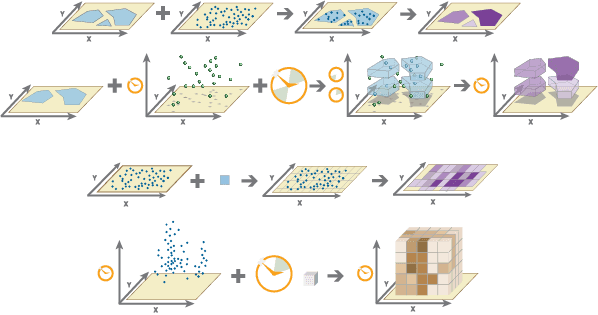## Usage notes

• Aggregate Points is designed to collect and summarize points within a set of boundaries. The input DataFrame must contain point geometries to be aggregated.

• There are two ways to specify the boundaries:

• Use a polygon DataFrame by specifying `setPolygons()`.
• Use a square, hexagonal or H3 bin of a specified size that is generated when the analysis is run by specifying `setBins()`.
• Specify bin shape using `setBins()`. The bin size specifies how large the bins are. If you are aggregating into hexagons, the size is the height of each hexagon, and the width of the resulting hexagon will be two times the height divided by the square root of three. If you are aggregating into squares, the bin size is the height of the square, which is equal to the width. If you are aggregating into H3 bins, you have two options. The first option is to set the bin height as the bin size. In this case, the bin resolution which is the closest to the bin height will be chosen. You can check the H3 document for more details on the resolution and edge lengths. The width of the resulting H3 bin will be the height divided by the square root of three. The second option is to set the H3 resolution as the bin size. For the parameter `bin_size` you can specify any resolution between 0-15 and set the `bin_size_unit` to `h3res`.• Analysis with hexagonal or square bins requires that your input DataFrame's geometry has a projected coordinate system. If your data is not in a projected coordinate system, the tool will transform your input to a World Cylindrical Equal Area (SRID: 54034) projection. You can transform your data to a projected coordinate system by using ST_Transform. Analysis with H3 bins requires that your input DataFrame's geometry is in the World Geodetic System 1984 coordinate system. If not, this transformation will be applied.

• The output is always a DataFrame with polygon geometries. Only polygons that contain points will be returned.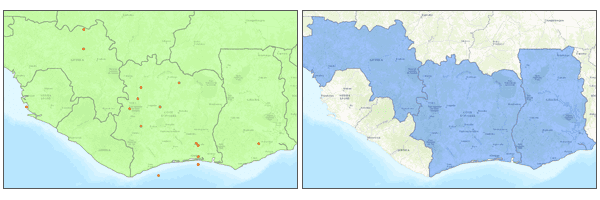• If time is enabled on the input DataFrame, you can apply time stepping to your analysis. Each time step is analyzed independent of points outside the time step. To use time stepping, your input data must be time enabled and represent an instant in time. When time stepping is applied, rows returned in the output DataFrame will have time type of interval represented by the `start_time` and `end_time` fields.

• The most basic aggregations will calculate a count of the number of points in each polygon. Statistics (count, sum, minimum, maximum, range, mean, standard deviation, and variance) can also be calculated on numerical fields. Count and any can be calculated on string fields. The statistics will be calculated on each area separately. If you specify a statistic that is not valid (mean of a string field), it will be skipped.

• When the count statistic is applied to a field, it returns a count of the nonnull values present in the field. When the any statistic is applied to a string field, it returns a single string present in the field.

## Limitations

• Input DataFrames must contain point geometries. The polygons to aggregate into must be a polygon DataFrame if bins are not used. Linestrings and polygons cannot be used as the input.
• Only the polygons that contain points are returned.

## Results

The tool outputs polygons that include the following fields:

FieldDescriptionNotes
`count`The count of input points within each polygon.
`<statistic>_<fieldname>`Specified statistics will each create an attribute field, named in the following format: statistic_fieldname. For example, the maximum and standard deviation of the `id` field are `MAX_id` and `SD_id`, respectively.
`step_start`When time stepping is specified, output polygons will have a time interval. This field represents the start time.
`step_end`When time stepping is specified, output polygons will have a time interval. This field represents the end time.
`bin_geometry`The geometry of the result bins if aggregating into bins.

## Performance notes

Improve the performance of Aggregate Points by doing one or more of the following:

• Only analyze the records in your area of interest. You can pick the records of interest by using one of the following SQL functions:

• ST_Intersection—Clip to an area of interest represented by a polygon. This will modify your input records.
• ST_EnvIntersects—Select records that intersect an envelope.
• ST_Intersects—Select records that intersect another dataset or area of intersect represented by a polygon.
• Larger bins will perform better than smaller bins. If you are unsure about which size to use, start with a larger bin to prototype.
• Similar to bins, larger time steps will perform better than smaller time steps.

Similar tools:

## How Aggregate Points works

### Equations

Variance is calculated using the following equation: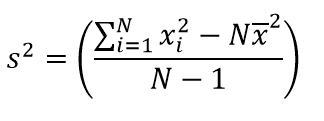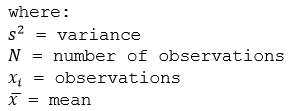Standard deviation is calculated as the square root of the variance.

### Calculations

• Points are summarized using only the points that are intersecting the input boundary.

• The following figure and table illustrate the statistical calculations of a point DataFrame within district boundaries. The `Population` field was used to calculate the statistics (Count, Sum, Minimum, Maximum, Range, Mean, Standard Deviation, and Variance) for the input DataFrame. The `Type` field was used to calculate the statistics (Count and Any) for the input DataFrame.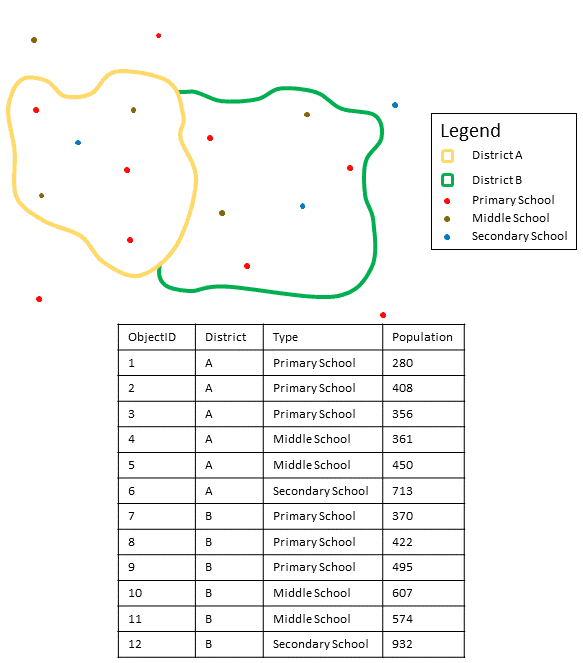An example attribute table is displayed above with values to be used in this hypothetical statistical calculation.

Numeric statisticResults District A
CountCount of: `[280, 408, 356, 361, 450, 713] = 6`
Sum`280 + 408 + 356 + 361 + 450 + 713 = 2568`
MinimumMinimum of: `[280, 408, 356, 361, 450, 713] = 280`
MaximumMaximum of: `[280, 408, 356, 361, 450, 713] = 713`
Average`2568/6 = 428`
Variance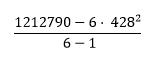`= 22737.2`
Standard Deviation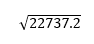`= 150.7886`
String statisticResults District A
Count`= 6`
Any`= Secondary School`
• The count statistic (for strings and numeric fields) counts the number of nonnull values. The count of the following values equals 5: `[0, 1, 10, 5, null, 6]` = `5`. The count of this set of values equals 3: `[Primary, Primary, Secondary, null]` = `3`.

• The first and last statistic is based on the temporal order of the records in each group.

## Syntax

For more details, go to the GeoAnalytics Engine API reference for aggregate points.

SetterDescriptionRequired
`run(dataframe)`Runs the Aggregate Points tool using the provided DataFrame.Yes
`addSummaryField(summary_field, statistic, alias=None)`Adds a summary statistic of a field in the input DataFrame to the result DataFrame.No
`setBins(bin_size, bin_size_unit, bin_type='square')`Sets the size and shape of bins used to aggregate points into.Either `setBins()` or `setPolygons()` is required.
`setPolygons(polygons)`Sets the polygons into which points will be aggregated.Either `setBins()` or `setPolygons()` is required.
`setTimeStep(interval_duration, interval_unit, repeat_duration=None, repeat_unit=None, reference_time=None)`Sets the time step interval, time step repeat, and reference time. If set, points will be aggregated into each bin for each time step.No

## Examples

### Run Aggregate Points

Python
Use dark colors for code blocksCopy
``````# Log in
import geoanalytics

# Imports
from geoanalytics.tools import AggregatePoints
from geoanalytics.sql import functions as ST
from pyspark.sql import functions as F

# Path to the earthquakes data
data_path = r"https://sampleserver6.arcgisonline.com/arcgis/rest/services/" \
"Earthquakes_Since1970/FeatureServer/0"

# Create an earthquakes DataFrame and transform the spatial reference
# to World Cylindrical Equal Area (54034)
.withColumn("shape", ST.transform("shape", 54034))

# Use Aggregate Points to summarize the count of world earthquakes into 10 year intervals
result = AggregatePoints() \
.setBins(bin_size=800, bin_size_unit="Kilometers", bin_type="Hexagon") \
.setTimeStep(interval_duration=10, interval_unit="years") \
.run(df)

# Show the 5 rows of the result DataFrame with the highest count
result = result.select("COUNT", "bin_geometry", "MIN_magnitude", "MAX_magnitude",
F.date_format("step_start", "yyyy-MM-dd").alias("step_start"),
F.date_format("step_end", "yyyy-MM-dd").alias("step_end"))
result.sort("COUNT", "MIN_magnitude", ascending=False).show(5)
``````
Result
Use dark colors for code blocksCopy
``````+-----+--------------------+-------------+-------------+----------+----------+
|COUNT|        bin_geometry|MIN_magnitude|MAX_magnitude|step_start|  step_end|
+-----+--------------------+-------------+-------------+----------+----------+
| 18.0|{"rings":[[[78519...|          4.7|          7.4|1999-12-31|2009-12-31|
| 15.0|{"rings":[[[85447...|          4.6|          7.5|1989-12-31|1999-12-31|
| 15.0|{"rings":[[[57735...|          4.5|          6.1|1999-12-31|2009-12-31|
| 14.0|{"rings":[[[30022...|          4.0|          7.5|1979-12-31|1989-12-31|
| 13.0|{"rings":[[[1.616...|          5.2|          7.2|1999-12-31|2009-12-31|
+-----+--------------------+-------------+-------------+----------+----------+
only showing top 5 rows``````

### Plot results

Python
Use dark colors for code blocksCopy
``````# Create a continents DataFrame and transform geometry to World Cylindrical
# Equal Area (54034) for plotting
continents_path = "https://services.arcgis.com/P3ePLMYs2RVChkJx/ArcGIS/rest/" \
"services/World_Continents/FeatureServer/0"
.withColumn("shape", ST.transform("shape", 54034))

# Plot the Aggregate Points result DataFrame for the time step interval
# of 12-31-1969 to 12-31-1979 with the world continents
continents_plot = continents_df.st.plot(facecolor="none",
edgecolors="lightblue")
result_plot1 = result.where("step_start = '1969-12-31'") \
.st.plot(cmap_values="COUNT",
cmap="YlOrRd",
figsize=(4,4),
legend=True,
legend_kwds={"orientation": "horizontal", "location": "bottom", "shrink": 0.8, "pad": 0.08},
ax=continents_plot,
vmin=0,
vmax=14,
basemap="light")
result_plot1.set_title("Aggregate count of world earthquakes from 12-31-1969 to 12-31-1979", {'fontsize': 8})
result_plot1.set_xlabel("X (Meters)")
result_plot1.set_ylabel("Y (Meters)");

# Plot the Aggregate Points result DataFrame for the time step interval
# of 12-31-1979 to 12-31-1989 with the world continents
continents_plot = continents_df.st.plot(facecolor="none",
edgecolors="lightblue")
result_plot2 = result.where("step_start = '1979-12-31'") \
.st.plot(cmap_values="COUNT",
cmap="YlOrRd",
figsize=(4,4),
legend=True,
legend_kwds={"orientation": "horizontal", "location": "bottom", "shrink": 0.8, "pad": 0.08},
ax=continents_plot,
vmin=0,
vmax=14,
basemap="light")
result_plot2.set_title("Aggregate count of world earthquakes from 12-31-1979 to 12-31-1989", {'fontsize': 8})
result_plot2.set_xlabel("X (Meters)")
result_plot2.set_ylabel("Y (Meters)");``````

ReleaseNotes

1.0.0

Tool introduced

1.2.0